### Python3对股票数据进行分析

1、量化交易（投资方法）

2、算法交易（自动交易、黑盒交易或机器交易）

3、量化策略

4、量化选股

5、股票回测

1、导入股票时间序列数据

2、绘制股票成交量的时间序列图

3、绘制股票收盘价和成交量的时间序列图

3、绘制K线图（蜡烛图）

（1）K线图理论

（2）K线图绘制

4、股票指标相关性分析

（1）相关关系分析

（2）相关系数（Correlation coefficient）分析

5、移动平均线

# 二、股票数据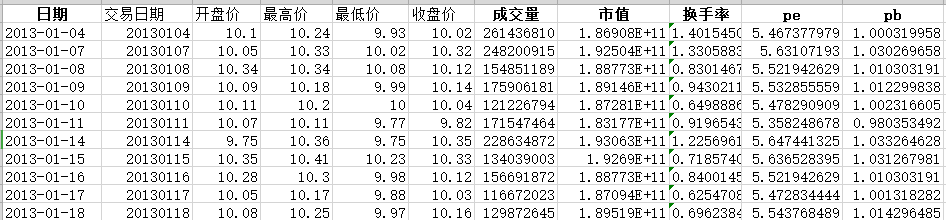股票指标名称 指标含义 开盘价（open） 每个交易日开市后的第一笔每股买卖成交价格 最高价（high） 最低价（low） 最高价是好的卖出价格，最低价是好的买进价格，可根据价格极差判断股价的波动程度和是否超出常态范围 收盘价（close） 最后一笔交易前一分钟所有交易的成交量加权平均价，无论当天股价如何振荡，最终将定格在收盘价上 成交量（volume） 指一个时间单位内对某项交易成交的数量，可根据成交量的增加幅度或减少幅度来判断股票趋势，预测市场供求关系和活跃程度 市值（market value） 市场价格总值，可以市值的增加幅度或减少幅度来衡量该种股票发行公司的经营状况 换手率（turnover） “换手率”也称“周转率”，指在一定时间内市场中股票转手买卖的频率，是反映股票流通性强弱的指标之一 pe 市盈率, 股票价格除以每股盈利的比率，评估股价水平是否合理的指标之一，反应企业近期表现，可根据其大小判断投资回收期的长短和投资风险大小 pb 市净率，股价除以账面价值，可衡量该种股票的投资价值和投资风险

# 三、股票数据分析

## 1、导入股票时间序列数据

``from pandas import read_excel## 读取excel文件，并将‘日期’列解析为日期时间格式,并设为索引stock_data=read_excel('stock_data/600000.SH.xlsx',parse_dates=['日期'],index_col='日期')stock_data.drop('交易日期', axis=1, inplace=True) #删除第二列’交易日期‘stock_data.index.name='日期' #日期为索引列#将数据按日期这一列排序（保证后续计算收益率的正确性）stock_data=stock_data.sort_values(by='日期')# 打印数据的前5行print(stock_data.head())``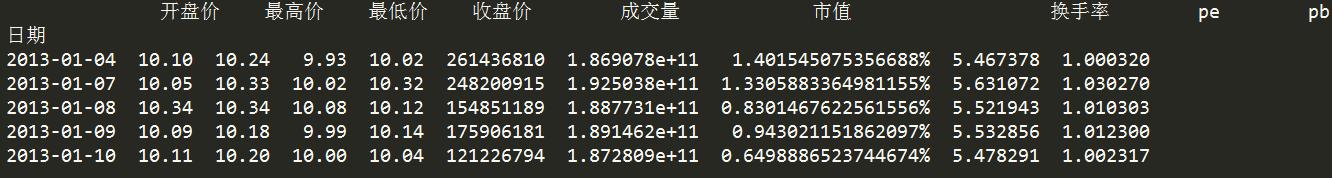``print(stock_data.info())``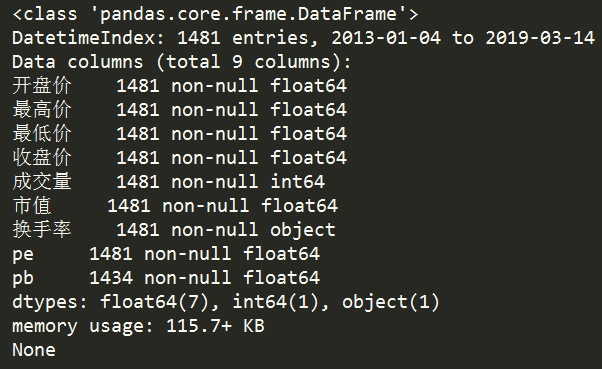## 2、绘制股票成交量的时间序列图

绘制股票在2013年到2019年的日成交量的时间序列图。

``import matplotlib.pyplot as pltstock_data['成交量'].plot(grid=True,color='red',label='600000.SH')plt.title('2013-2019 volume', fontsize='9')plt.ylabel('volume', fontsize='8')plt.xlabel('date', fontsize='8')plt.legend(loc='best',fontsize='small')plt.show()``

结果为：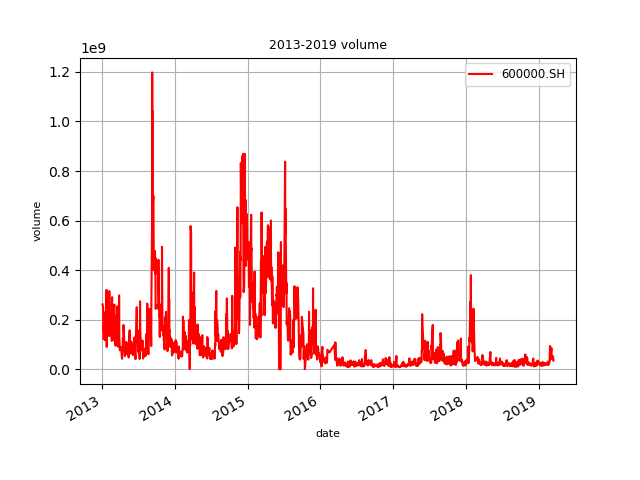## 3、绘制股票收盘价和成交量的时间序列图

``stock_data.index.name='date' #日期为索引列#对股票数据的列名重新命名stock_data.columns=['open','high','low','close','volume','market_value','turnover','pe','pb']data=stock_data.loc['2016-02-15':'2017-12-29']  #获取某个时间段内的时间序列数据data[['close','volume']].plot(secondary_y='volume',grid=True)plt.title('2016-2017 close and volume', fontsize='9')plt.show()``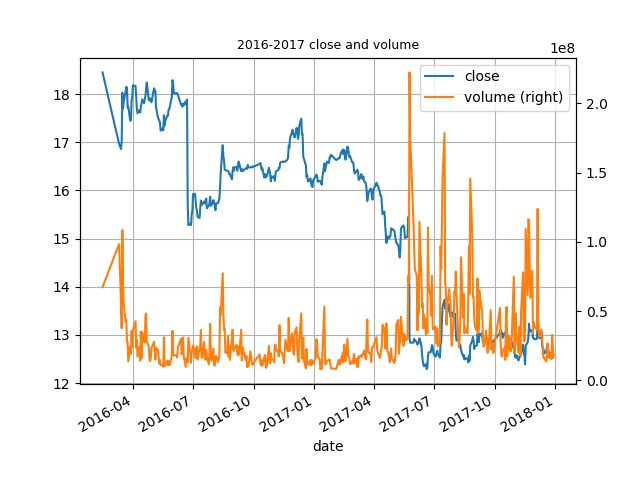## 3、绘制K线图（蜡烛图）

### （1）K线图理论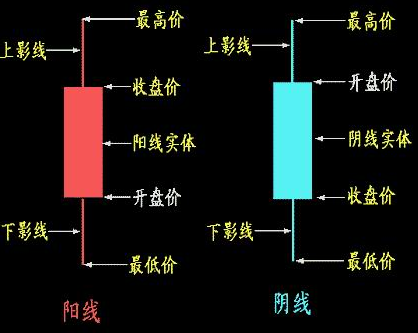### （2）K线图绘制

绘制股票在2013年3月份每日的开盘价，最高价，最低价，收盘价的K线图。

``import numpy as npfrom mpl_finance import candlestick_ohlcfrom matplotlib.dates import DateFormatter, WeekdayLocator, DayLocator, MONDAY,date2num #定义绘制K线图的函数def pandas_candlestick_ohlc(stock_data, otherseries=None):    # 设置绘图参数，主要是坐标轴    mondays = WeekdayLocator(MONDAY)    alldays = DayLocator()    dayFormatter = DateFormatter('%d')     fig, ax = plt.subplots()    fig.subplots_adjust(bottom=0.2)    if stock_data.index[-1] - stock_data.index < pd.Timedelta('730 days'):        weekFormatter = DateFormatter('%b %d')        ax.xaxis.set_major_locator(mondays)        ax.xaxis.set_minor_locator(alldays)    else:        weekFormatter = DateFormatter('%b %d, %Y')    ax.xaxis.set_major_formatter(weekFormatter)    ax.grid(True)     # 创建K线图    stock_array = np.array(stock_data.reset_index()[['date','open','high','low','close']])    stock_array[:,0] = date2num(stock_array[:,0])    candlestick_ohlc(ax, stock_array, colorup = "red", colordown="green", width=0.6)     # 可同时绘制其他折线图    if otherseries is not None:        for each in otherseries:            plt.plot(stock_data[each], label=each)        plt.legend()     ax.xaxis_date()    ax.autoscale_view()    plt.setp(plt.gca().get_xticklabels(), rotation=45, horizontalalignment='right')    plt.show() stock_data.index.name='date' #日期为索引列#对股票数据的列名重新命名stock_data.columns=['open','high','low','close','volume','market_value','turnover','pe','pb']data=stock_data.loc['2016-02-15':'2016-03-31']  #获取某个时间段内的时间序列数据pandas_candlestick_ohlc(data)``

结果为：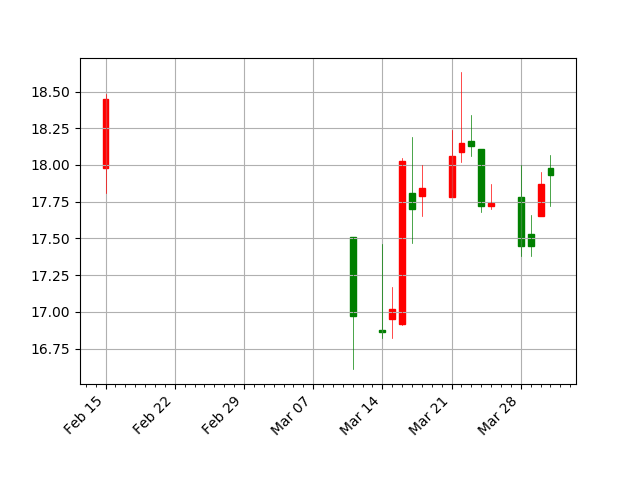该图红色代表上涨，绿色代表下跌。

## 4、股票指标相关性分析

### （1）相关关系分析

``from pandas.plotting import scatter_matrix#换手率字符串类型转换为浮点型函数def str_to_float(s):    s=s[:-1]    s_float=float(s)    return s_floatstock_data['换手率']=stock_data['换手率'].apply(str_to_float)#换手率字符串类型转换为浮点型#对股票数据的列名重新命名stock_data.columns=['open','high','low','close','volume','market_value','turnover','pe','pb']data=stock_data.loc['2018-01-02':'2018-12-28']  #获取某个时间段内的时间序列数据scatter_matrix(data[['volume','market_value','turnover','pe','pb']])plt.show()``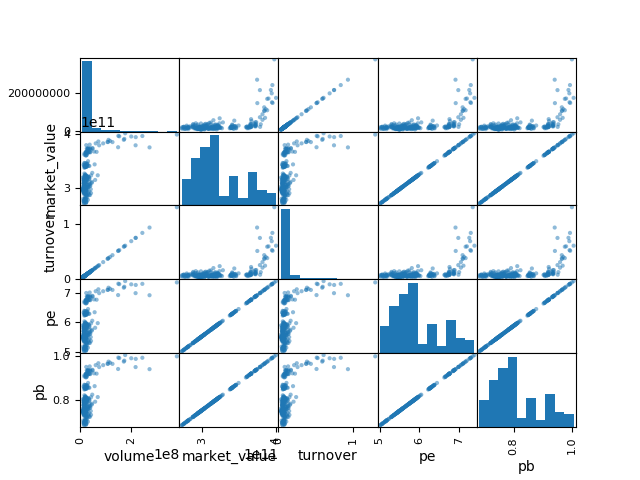### （2）相关系数（Correlation coefficient）分析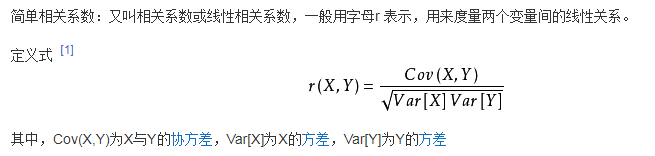``#换手率字符串类型转换为浮点型函数def str_to_float(s):    s=s[:-1]    s_float=float(s)    return s_floatstock_data['换手率']=stock_data['换手率'].apply(str_to_float)#换手率字符串类型转换为浮点型#对股票数据的列名重新命名stock_data.columns=['open','high','low','close','volume','market_value','turnover','pe','pb']data=stock_data.loc['2018-01-02':'2018-12-28']  #获取某个时间段内的时间序列数据cov=np.corrcoef(data[['volume','market_value','turnover','pe','pb']].T)print(cov)``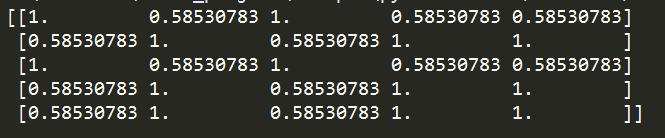``cov=np.corrcoef(data[['volume','market_value','turnover','pe','pb']].T)img=plt.matshow(cov)plt.colorbar(img,ticks=[-1,0,1])plt.show()``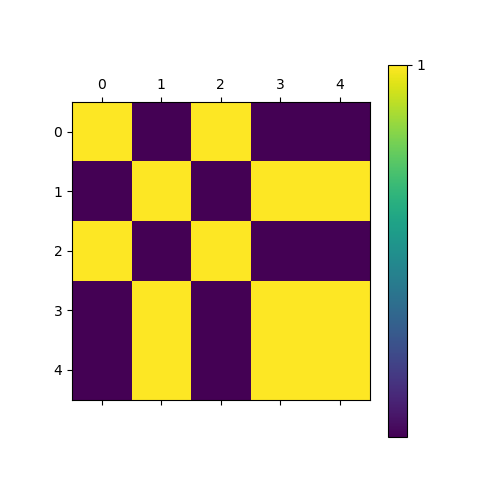## 5、移动平均线

``stock_data['close_mean5']=np.round(stock_data['close'].rolling(window=5,center=False).mean(),2)stock_data['close_mean20']=np.round(stock_data['close'].rolling(window=20,center=False).mean(),2)data=stock_data.loc['2016-03-11':'2016-05-31']  #获取某个时间段内的时间序列数据pandas_candlestick_ohlc(data,['close_mean5','close_mean20'])``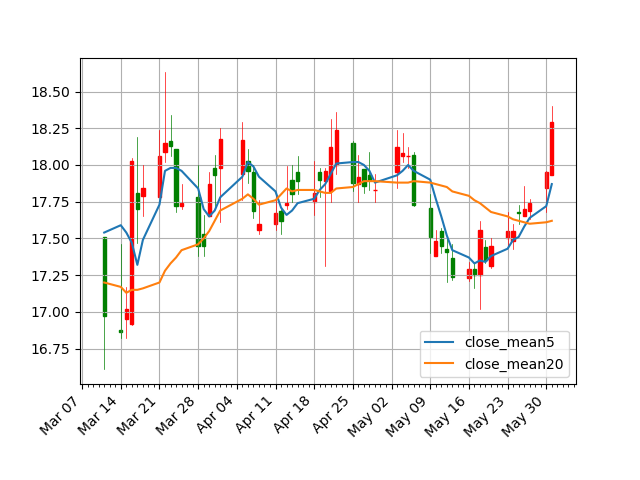``stock_data['close_m5-20']=stock_data['close_mean5']-stock_data['close_mean20']stock_data['diff']=np.sign(stock_data['close_m5-20'])data=stock_data.loc['2016-03-11':'2016-05-31']  #获取某个时间段内的时间序列数据data['diff'].plot(ylim=(-2,2)).axhline(y=0,color='black',lw=2)plt.show()``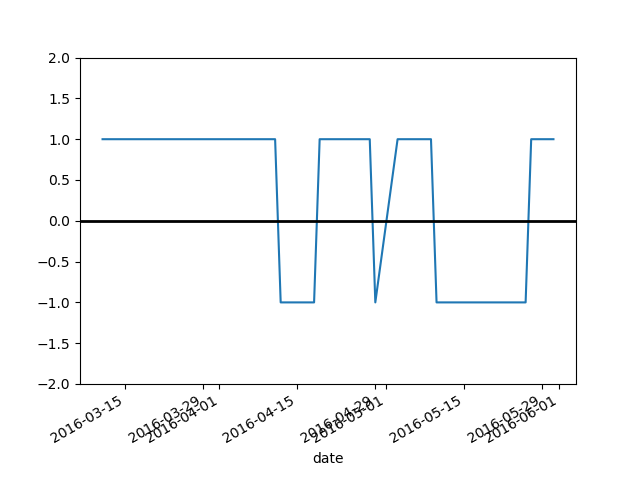``data['signal'] = np.sign(data['diff'] - data['diff'].shift(1))data['signal'].plot(ylim=(-2,2))plt.show()``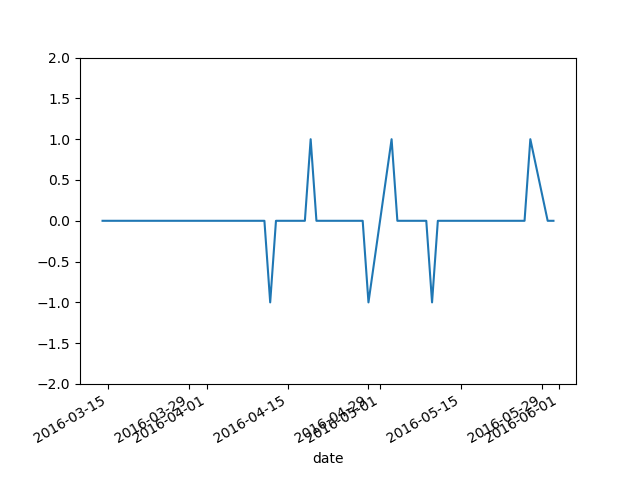``trade = pd.concat([  pd.DataFrame({"price": data.loc[data["signal"] == 1, "close"],"operation": "Buy"}),                     pd.DataFrame({"price": data.loc[data["signal"] == -1, "close"],"operation": "Sell"})  ])trade.sort_index(inplace=True)print(trade)``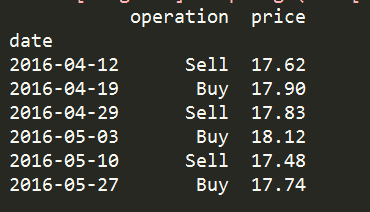Python3对股票数据进行分析源代码和股票数据集资源下载：

Python3对股票数据进行分析源代码和股票数据集-机器学习文档类资源-CSDN下载

1、基于移动平均的交易策略

2、数据分析实践之路

1、Python3对股票数据进行分析（项目实战源代码和股票数据资源下载）

2、Python3对股票的收益和风险进行分析（项目实战源代码和股票数据资源下载）

3、LSTM对股票的收益进行预测（Keras实现）（项目实战源代码和股票数据资源下载）

4、Python3对多股票的投资组合进行分析（项目实战源代码和股票数据资源下载​​​​​​​）